## Chapter 4: FINDINGS AND ANALYSIS

### Introduction

In this chapter of the study, the researcher has presented the findings and results. This research has been carried out to analyse the relationship and impact between corporate distress, corporate social responsibility, and firm life cycle with respect to the evidence from Pakistan. To find out the aim of the research the researcher has collected secondary quantitative data from 150 Pakistani firms for the time period extending from 2006 to 2016. The data for the dependent variable that is financial distress within the company has been collected with the help of Altman’s Z score and the data for an independent variable that is corporate social responsibility performance has been collected with the help of social investment. Moreover, another independent variable of this study is the firm’s lifecycle which is measured with the help of a five-stage life cycle model proposed by Dickinson. In order to support the model, there are also certain control variables which are used by the researcher: the size of the firm, leverage, cash holdings, ROA, cash plus receivables divided by current liabilities, and two dummy variables. In order to evaluate the results, the researcher has used Ordinary Least Squares (OLS) regression. Moreover, the relationship has been found with the help of correlation analysis. The concluding part of this chapter is a discussion where the researcher has related the findings of this research with the objectives. Moreover, in the discussion section, the findings of this study are also linked to the previous studies which are carried out in this domain.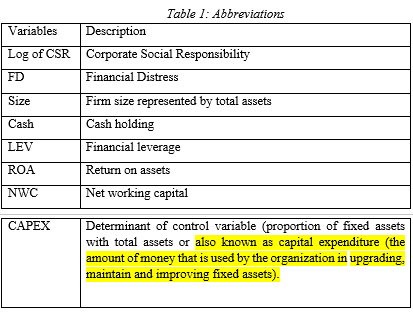### Descriptive Analysis

For the purpose of finding out the general characteristics of the data that has been used for this research, descriptive statistics have been used. According to George and Mallery (2016), descriptive statistics allow the researcher to summarise the statistical characteristics and `specificities of the sample used for the study. For this study, the researcher has focused on two components of descriptive statistics: (1) measures of central tendency and (2) measures of variation. Following are the results that are obtained from Stata regarding the descriptive statistics of the overall firms: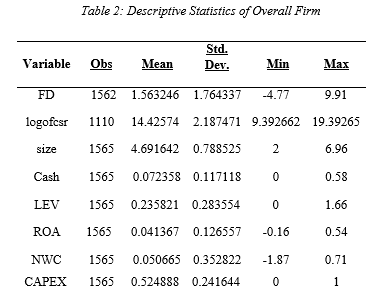There are 8 variables that are involved in the following study in which the logofcsr represents the natural log for total investments of firms in CSR. The FD represents the financial distress in which Altman’s Z score had been used as proxy. ROA represents return on assets in which it had been measured through ratio of net income and total assets. The size indicates the total assets of the firm. The variable cash represents the total cash holding of the company. NWC (Net working capital) is the difference between the current assets and current liabilities of the firm. And lastly CAPEX represents the determinant of control variable (proportion of fixed assets with total assets.

The above table shows the total observation for each value, their average values represented by mean, variation in the mean represented by standard deviation, the minimum value of the variable in the entire dataset, and maximum value of the variable in the entire dataset. The variable of FD is the determinant of the financial score and the average value shown by the mean is calculated to be 1.563. According to the standard deviation value for the variable, the data set is dispersed by 1.764 units. The minimum financial distress recorded in the data set is -4.77 and the maximum is recorded to be 9.91. The variable of size represents the size of the firms and the average value shown by the mean is calculated to be 4.69. According to the standard deviation value for the variable, the data set is dispersed by 0.788 units. The minimum size of the firms recorded in the data set is 2 and the maximum is recorded to be 6.96. The variable of Cash represents cash and the average value shown by the mean is calculated to be

0.072. According to the standard deviation value for the variable, the data set is dispersed by 0.117 units. The minimum cash (Cash) recorded in the data set is 0 and the maximum is recorded to be 0.58. The variable of LEV represents the total leverage of the firm and the average value shown by the mean is calculated to be 0.235. According to the standard deviation value for the variable, the data set is dispersed by 0.283 units. The minimum leverage recorded in the data set is 0 and the maximum is recorded to be 1.66. The average value for the variable ROA is calculated to be 0.041. According to the standard deviation value for the variable, the data set is dispersed by 0.126 units. The minimum ROA recorded in the data set is -0.16 and the maximum is recorded to be 0.54. The variable of CAPEX is the determinant of the control variable (proportion of fixed assets with total assets) and the average value shown by the mean is calculated to be 0.524. According to the standard deviation value for the variable, the data set is dispersed by 0.24 units. The minimum financial distress recorded in the data set is 0 and the maximum is recorded to be 1.

### Descriptive Analysis across Life Cycle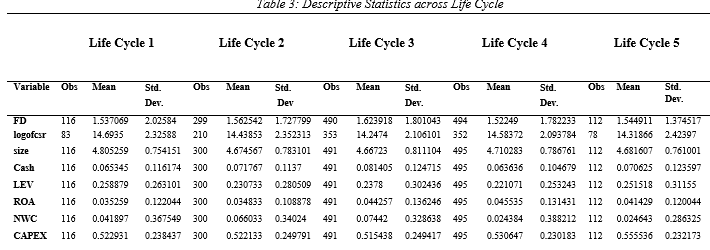There are 8 variables that are involved in the following study in which the logofcsr represents the natural log for total investments of firms in CSR. The FD represents the financial distress in which Altman’s Z score had been used as proxy. ROA represents return on assets in which it had been measured through ratio of net income and total assets. The size indicates the total assets of the firm. The variable cash represents the total cash holding of the company. NWC (Net working capital) is the difference between the current assets and current liabilities of the firm. And lastly CAPEX represents the determinant of control variable (proportion of fixed assets with total assets). The above table indicates the descriptive analysis across the lifecycle in which there is 5 life cycle.

Lifecycle 1 indicates the introduction stage, lifecycle 2 is the growth stage, lifecycle 3 represents the maturity stage, lifecycle 4 highlights the shake off stage and the lifecycle 5 indicates the decline stage. The mean of financial stress (FD) in lifecycle 1 (introduction) is calculated to be 1.53. The corporate social responsibility (logofcsr) had an average mean of 14.69 in lifecycle one. The firm assets which are represented by size had an average mean of

4.80 whereas the cash mean in the lifecycle 1 was calculated to be 0.06. The return on assets (ROA) had an average mean of 0.03 in lifecycle 1 and networking capital had an average mean of (0.041). The variable of CAPEX is the determinant of the control variable (proportion of fixed assets with total assets) and the average value shown by the mean is calculated to be 0.52 in the lifecycle 1.

The mean of financial stress (FD) in lifecycle 2 (growth) is determined to be 1.56. The corporate social responsibility had an average mean of 14.43 in the growth lifecycle. The firm assets (sized) average mean had been calculated as 4.67 whereas the cash had a mean in the lifecycle 2 was calculated to be 0.71. The return on assets had an average mean of 0.03 in lifecycle 2 and networking capital had an average mean of (0.041). The variable of CAPEX is the determinant of Proportion of fixed assets and the average value shown by the mean is calculated to be 0.52 in the lifecycle 2 (growth).

Furthermore, in the lifecycle 3 (maturity), the mean of the financial distress was 1.62 which had been increased in the maturity phase. The mean of CSR had almost remained the same as it had been calculated as 14.24, the firm assets (size) had the mean of 4.66 which had been decreasing from the introduction phase. The mean of cash holding was 0.08 which had been increasing from the introduction phase, the average mean of financial leverage had been calculated as 0.23 and the return on assets mean was 0.04. The mean of net working capital was 0.07 and lastly, the mean of the proportion of fixed assets had been decreasing form maturity stage which was 0.51.

In the Lifecycle 4 (Shake-Out phase), the mean of financial distress had decreased to 1.52 from the maturity phase; the mean of CSR had slightly increased in this phase to 14.58. Similarly, the size of the firm assets had also increased to 4.71, the mean of cash holding had decreased to 0.063 and financial leverage mean had decreased to 0.22. The return on assets had the average mean of 0.045 which increased slightly from the maturity phase and net working capital had significantly reduced in this phased to 0.02.

In the lifecycle phase 5 (Decline), the mean of financial distress was 1.544 which had increased, CSR average mean had decreased in this phase to 14.31. The firm size had an average mean of 4.68 which had slightly decreased from the shake off phase; the mean of cash holding had increased in this phase to 0.07. The mean of financial leverage had increased to 0.25 whereas the return on asset mean had decreased to 0.041. The net working capital had an average mean of 0.24 which had remained the same and the proportion of fixed assets had increased in this phase to 0.55.

### Correlation Analysis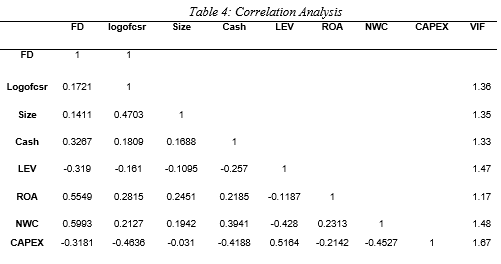There are 8 variables that are involved in the following study in which the logofcsr represents the natural log for total investments of firms in CSR. The FD represents the financial distress in which Altman’s Z score had been used as proxy. ROA represents return on assets in which it had been measured through ratio of net income and total assets. The size indicates the total assets of the firm. The variable cash represents the total cash holding of the company. NWC (Net working capital) is the difference between the current assets and current liabilities of the firm. And lastly CAPEX represents the determinant of control variable (proportion of fixed assets with total assets). .” Note; *p<.05 (significant at 95%); **p<.01 (significant at 99%); ***p<.001 (significant at 99.9%); and ***Highly Significant .00, **Significant .01 and *Poorly Significant .05.

The correlation analysis had been conducted through the Pearson correlation in which the values in this table are the coefficients. According to (Cohen, West, & Aiken, 2014) the coefficients are analyzed for understanding the variable’s relationship with each other. If the coefficient has a negative sign then this indicates a negative relationship whereas coefficient without a negative sign would indicate a positive relationship. Furthermore, the study had indicated that if the coefficient is near 0 and is less than 0.5 would indicate a weak positive relation whereas the coefficient lying between 0.5 – 0.7 would indicate a moderate positive relationship and lastly, coefficient which is closer than 1 and is above 0.7 would highlight a strong positive relationship.

Since the dependent and the main variable in this study is the financial distress which is represented by FD; therefore, its coefficient with other variables had been considered for analysing and interpreting the results. The correlation coefficient of FD with the logofcsr, size and cash is 0.17, 0.14 and 0.32, respectively. As the coefficients are near to 0, thus, it can be stated that the relationship of financial distress with the corporate social responsibility, firm size and cash holding is a positive and weak relationship. Therefore, it indicates that the positive change on these three variables would also bring a change to the financial distress; however, the change would be lower as well as significant relating to its relationship.

Moving on, the correlation coefficient of financial distress with the financial leverage and Proportion of fixed assets is -0.319 and -0.31 which indicates a weak negative relationship. This indicates that the increase of financial distress would bring a negative change on the financial leverage and proportion of fixed assets. Other than that, the financial distress had 0.55 and 0.59 coefficient with the return on assets and net working capital, respectively. It can be interpreted that the return on assets and NWC has a moderate positive relation in which the change on these two variables would also bring a moderate positive change to the financial distress. Based on the above analysis of correlation, it can be concluded that financial distress has a positive and weak relationship with CSR, firm size and cash holding whereas it has a positive and moderate relationship with return on assets and net working capital. On the other hand, the variables financial leverage and the proportion of fixed assets had a negative and weak relationship.

Other than that coefficients, the variance inflation factor or also referred to as VIF had been analyzed that supports in detecting the multicollinearity. The multicollinearity occurs when there is a correlation between the independent variable which can cause adverse effect to the regression analysis. The VIF ranges upwards from 1 in which if it is higher than 10 can indicate a high correlation which causes concern for regression (Lin, Dongyu, Foster, & Ungar, 2011)Similarly, from the above table, the mean of the VIF is 1.40 which is lower than 10. Therefore, this indicates that there is no multicollinearity in the data set which shows that there will be no adverse impact on the regression analysis.

### Regression Analysis

#### Regression Analysis (Overall)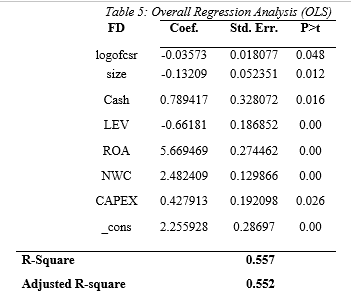There are 8 variables that are involved in the following study in which the logofcsr represents the natural log for total investments of firms in CSR. The FD represents the financial distress in which Altman’s Z score had been used as proxy. ROA represents return on assets in which it had been measured through ratio of net income and total assets. The size indicates the total assets of the firm. The variable cash represents the total cash holding of the company. NWC (Net working capital) is the difference between the current assets and current liabilities of the firm. And lastly CAPEX represents the determinant of control variable (proportion of fixed assets with total assets). Note; *p<.05 (significant at 95%); **p<.01 (significant at 99%); ***p<.001 (significant at 99.9%); and ***Highly Significant .00, **Significant .01 and *Poorly Significant .05

The above table represents the summarized results of the regression analysis in which the total number of observation that is made in this model is 1048 whereas the R-square of the pooled OLS (ordinary least squares) is 0.557 or 55.70% that indicates that how well the data is fit with the line of regression and what portion of the dependent variable can understand or analysed through independent variables. Since the R-square is 55.70%, this shows that the financial distress can be explained by 55.70% by the other independent variables. All the independent variable had been found significant with the dependent variables as its P-value was lower than 0.05. The variable that would bring negative change to the financial distress is the corporate social responsibility, firm size and financial leverage by -0.03, -0.13 and -0.66 with every unit changes in these variables, respectively. Therefore, CSR, firm assets and financial leverage would decrease the financial distress among the companies. On the other hand with the every unit change on cash holdings, return on assets, net working capital and proportion of fixed assets with total assets will bring a positive change of 0.78, 5.66, 2.48 and 0.42 to the financial distress, respectively.

#### Regression Analysis Fixed Effects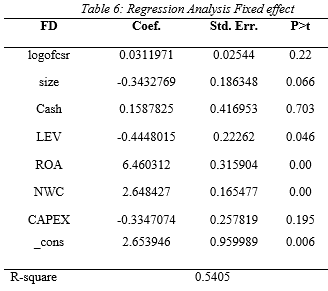There are 8 variables that are involved in the following study in which the logofcsr represents the natural log for total investments of firms in CSR. The FD represents the financial distress in which Altman’s Z score had been used as proxy. ROA represents return on assets in which it had been measured through ratio of net income and total assets. The size indicates the total assets of the firm. The variable cash represents the total cash holding of the company. NWC (Net working capital) is the difference between the current assets and current liabilities of the firm. And lastly CAPEX represents the determinant of control variable (proportion of fixed assets with total assets). .” Note; *p<.05 (significant at 95%); **p<.01 (significant at 99%); ***p<.001 (significant at 99.9%); and ***Highly Significant .00, **Significant .01 and *Poorly Significant .05

Similarly to the pooled regression OLS, the fixed effect regression analysis had been conducted on the data gathered. The data may be suffering for various problems that can cause invalid results to the regression analysis such as the elements serial correlation, heteroscedasticity and multicollinearity. Thus, the researcher had decided to generation regression analysed with fixed effects to avoid the problems and obtaining better results.  Based on the analysis, the r-square value was 0.54 or 54.05% which indicates that the financial distress can be explained and interpreted by the independent variables by 54.05%. Other than that, the variables that had been found to be significant with the financial distress are the total assets, financial leverage, return on assets and net working capital due to having a p-value less than 0.05. This shows that with every change on total assets, financial leverage, return on assets and net working capital will bring a change of -0.34, -0.44, 6.56 and 2.64 to the financial distress, respectively. Thus, the total assets of the firm and financial leverage would reduce the financial distress of the company. On the other hand, the variables that had been found insignificant are the corporate social responsibility, cash holdings and proportion of fixed assets as its p-value was higher than 0.05.

#### Regression Analysis for overall firms lifecycle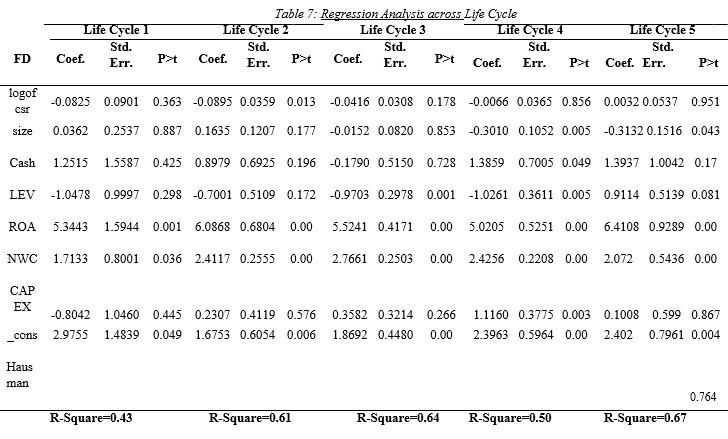There are 8 variables that are involved in the following study in which the logofcsr represents the natural log for total investments of firms in CSR. The FD represents the financial distress in which Altman’s Z score had been used as proxy. ROA represents return on assets in which it had been measured through ratio of net income and total assets. The size indicates the total assets of the firm. The variable cash represents the total cash holding of the company. NWC (Net working capital) is the difference between the current assets and current liabilities of the firm. And lastly CAPEX represents the determinant of control variable (proportion of fixed assets with total assets). .” Note; *p<.05 (significant at 95%); **p<.01 (significant at 99%); ***p<.001 (significant at 99.9%); and ***Highly Significant .00, **Significant .01 and *Poorly Significant .05

Table 7 represents the regression analysis (OLS) for the overall firm lifecycle in which there are five stages in the life cycle as discussed by Dickinson. The first stage is the introduction stage (Lifecycle 1), the second stage is growth stage (Lifecycle 2), the third stage is maturity stage (Lifecycle 3), the fourth stage is shake-out stage (Lifecycle 4) and decline stage (Lifecycle 5) is the fifth stage. The regression analysis had been conducted on each of the lifecycles for determining the impact on financial through the independent variables. The R-square in the lifecycle 1 was 0.45 or 45% which indicates that the financial distress can be understood or explained by 45% through the independent variable. The variables that had been found significant having p-value with less than 0.05 returned on assets and net working capital. Therefore, with every change unit to the return on assets and net working capital would bring a change of 5.34 and 1.71, respectively. Moreover, in the lifecycle 1, the CSR had an insignificant impact on financial distress due to its p-value being more than 0.05.

Moving further, the R-square of the lifecycle 2 was 0.61 meaning that the independent variable is able to explain or interpret the dependent variable by 61%. Other than that, the variables that had been significant were corporate social responsibility, ROA and NWC due to pvalues being less than 0.05. The CSR and financial distress had been associated in the growth phase which indicates that the firm mostly focuses on the CSR activities during the growth phase. The purpose is to create awareness among the public as well as attracting shareholders to the business. With every change in units to CSR will bring a negative change to the financial distress by -0.08. Similarly, in the maturity phase (Lifecycle 3), the variables that are significant with the financial distress are the financial leverage, return on assets and net working capital. With every change of units on financial leverage, return on assets and net working capital will bring a change of -0.97, 5.52 and 2.77 to the financial distress, respectively.

The variables that had been found significant on the shake-out stage (Lifecycle 4) are the total assets, cash holdings, financial leverage, return on assets, net working capital according and proportion of fixed assets according to its p-value. With every unit change on total assets, cash holding, financial leverage, return on assets, net working capital and proportion of fixed assets will bring a change of -0.30, 1.38, -1.02, 5.02 and 2.42 to the financial distress, respectively. Lastly, in the lifecycle 5 (Decline phase), the significant variables are the firm total asset, financial leverage, return on assets and net working capital. With every unit change to firm total asset, financial leverage, return on assets and net working capital will bring a change to financial distress by -0.31, 0.91, 6.41 and 2.07, respectively. The variables that had been found to remain significant throughout the lifecycle return on assets and net working capital.

#### Regression Analysis for overall firm’s lifecycle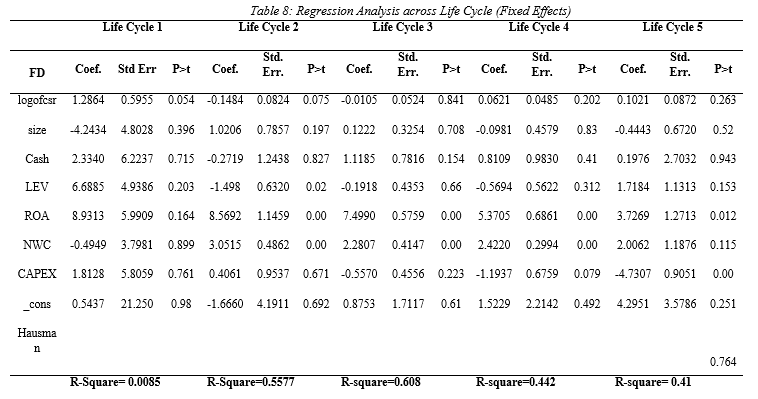There are 8 variables that are involved in the following study in which the logofcsr represents the natural log for total investments of firms in CSR. The FD represents the financial distress in which Altman’s Z score had been used as proxy. ROA represents return on assets in which it had been measured through ratio of net income and total assets. The size indicates the total assets of the firm. The variable cash represents the total cash holding of the company. NWC (Net working capital) is the difference between the current assets and current liabilities of the firm. And lastly CAPEX represents the determinant of control variable (proportion of fixed assets with total assets). .” Note; *p<.05 (significant at 95%); **p<.01 (significant at 99%); ***p<.001 (significant at 99.9%); and ***Highly Significant .00, **Significant .01 and *Poorly Significant .05

Table 8 represents the regression analysis (Fixed effects) for the overall firm lifecycle. The fixed effect model had been generated by keeping the parameters fixed. The results of the regression analysis in Lifecycle 1 were that there were no variables there significant with the financial distress in the introduction phase. In Lifecycle 2 (Growth phase), the significant variables were financial leverage, return on assets and net working capital. Moreover, the Rsquare was 0.55, meaning that the dependent variable is explained and interpreted by the independent variable by 55%. With every unit change on financial leverage, return on assets and net working capital would bring a -1.49, 8.56 and 3.05 change to financial distress.

In the Lifecycle 3 (Maturity), the R-square was 0.61 or 61% meaning that the independent variables are able to explain and interpret financial distress by 61%. Only two variables were significant in this stage which is a return on assets and net working capital. With every changing unit on return on assets and net working capital will bring a change of 7.49 and 2.28, respectively. In Lifecycle 4 (Shake-out), the R-square was 0.44 which indicates the dependent variable is explained and interpreted by 44% through the independent variable.  Moreover, the variables that had been identified significant in lifecycle 4 return on assets and net working capital due to p-value being less than 0.05. The change of every unit in ROA and NWC will cause a change of 5.37 and 2.42 to financial distress, respectively.

In the last lifecycle 5 (decline stage), the R-square value was 0.41 or 41% which indicates that the financial distress is explained by 41% through the independent variables. The significant variables are returned on assets and the proportion of fixed assets in the decline stage. With every change on units on return on assets and the proportion of fixed assets will cause 3.72 and 4.73 change to the financial distress.

### Relationship between Financial Distress and CSR

The first hypothesis that had been formulated by the researcher was to whether corporate social responsibility has a relationship with financial stress. From the regression analysis, it had been found that the corporate social responsibility had caused a negative influence on financial stress while also having significant value. Moreover, many of the companies conduct corporate social responsibility for the purpose of creating brand awareness and attracting shareholders and investors. This overall supports in gaining better financial results that ultimately helps the companies in repaying their loan and credits. The results gained from the correlation analysis had indicated that CSR has a positive and weak relationship with the financial distress. The reason was due to log of CSR which represents corporate social responsibility had coefficient of 0.1721. Thus, the H11 which states that the CSR has a significant relationship with financial distress had been accepted.

Similarly, the study of Godfrey (2009) matches with the results as it had been noted that the organizations and companies focus on the CSR activities for improving the societies and communities. The major objective of the CSR activities is to generate goodwill and capital which helps in times of financial distress. It also helps in attracting the stakeholders such as the shareholders for investing in the company. Moreover, the study of Attig (2013) had indicated a strong positive relationship among social performance and increased credit rating. The activities of CSR help the firms in creating a strong relationship with the stakeholders that overall support in cost reduction and effective performance on financials. Thus, the result gained from the analysis and also the review of the literature had indicated that CSR and financial distress are related as the activities of CSR can help in reducing the financial distress.

The second hypothesis of the study was to analyse the effect of the life cycle on the relationship between corporate social responsibility and financial distress. Dickinson (2011) had indicated five-stage life cycles which consist of introduction, growth, maturity, shake-out and decline. Based on the results, it had been found that the CSR affects the financial distress on the second stage of lifecycle which is the growth stage. The reason is that the variable CSR had significant impact having a p-value of less than 0.05 and also caused a negative impact on the financial distress. Therefore, it can be indicated that the companies focus on the CSR activities for the purpose of reducing financial distress especially on the second phase of lifecycle which is the growth stage. From the regression analysis results, it had been found that logofcsr had value less than 0.013 in the second stage of the lifecycle which is the growth stage whereas in the other stages CSR did not impact financial distress.. Thus, H21 had been accepted which states that the lifecycle has a significant relationship on the impact of CSR and financial distress.

The results also match with the study of Hasan (2017), in which the firm focuses on CSR for reducing the financial distress and also depends on the lifecycle. Other than that, the study of Edward (2013) had also indicated that the CSR activities help the business in forming a way out from the trap of financial distress. Moreover, the results of the study had further indicated that the CSR is also effective during the growing phase whereas in the later stages of the lifecycle is not effective with financial distress.

Related Samples